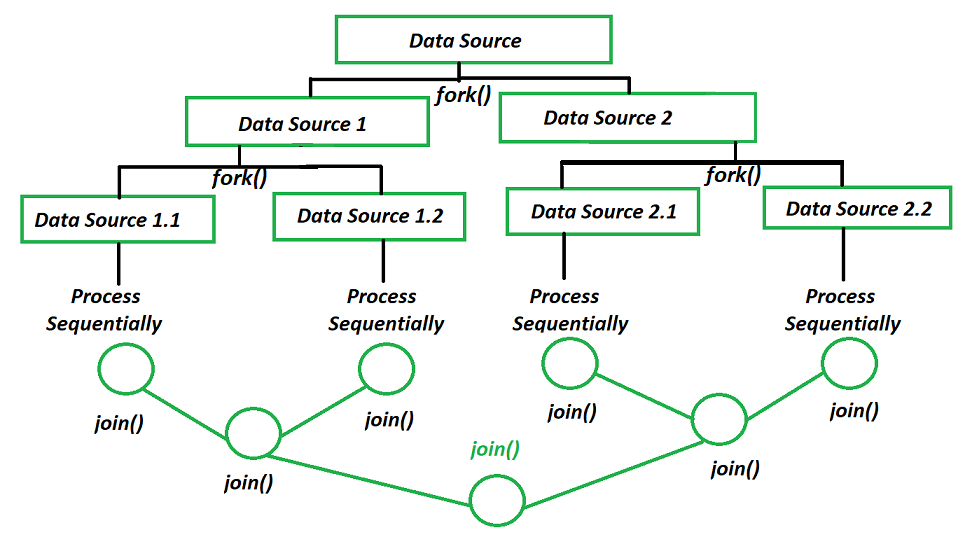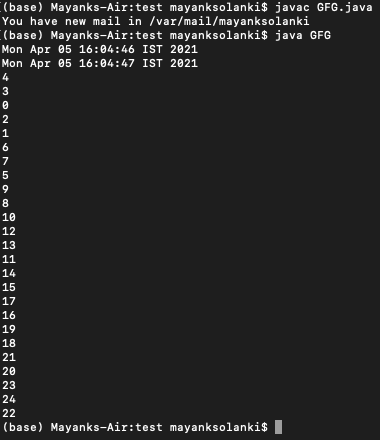# Difference Between Fork/Join Framework and ExecutorService in Java

• Last Updated : 01 Sep, 2021

The Fork/Join framework provides fine-grained task execution framework with high performance for Java data parallelism. Its parallel computing engine is used by many higher-level frameworks. The fork/join framework supports a style of parallel programming that solves problems by “Divide and conquer”, in the following manner as shown below:

• Sub-tasks can run in parallel on different cores.
• Sub-tasks can also run concurrently in different threads on a single core.
3. Waiting for them to complete
• join() waits for a sub-task to finish
4. Merging the results.
• A task uses calls to join() to merge the sub-task results together.Java Fork-Join Pool Computation Model

If a task does not return a result then it just waits for its sub-tasks to complete.

Attention reader! Don’t stop learning now. Get hold of all the important Java Foundation and Collections concepts with the Fundamentals of Java and Java Collections Course at a student-friendly price and become industry ready. To complete your preparation from learning a language to DS Algo and many more,  please refer Complete Interview Preparation Course.

Below is a Java program to demonstrate the working of Fork/Join Framework :

## Java

 `// Java program to demonstrate the working of Fork/Join``// Framework` `// Importing required libraries``import` `java.io.*;``import` `java.util.concurrent.ForkJoinPool;``import` `java.util.concurrent.RecursiveTask;` `// Class 1``// helper class``class` `SearchTask ``extends` `RecursiveTask {` `    ``// Global variables``    ``int` `array[];``    ``int` `start, end;``    ``int` `searchElement;` `    ``// Constructor for initialising globals``    ``public` `SearchTask(``int` `array[], ``int` `start, ``int` `end,``                      ``int` `searchElement)``    ``{` `        ``// This keyword regers to current object itself``        ``this``.array = array;``        ``this``.start = start;``        ``this``.end = end;``        ``this``.searchElement = searchElement;``    ``}` `    ``// Method``    ``// @Override``    ``protected` `Integer compute()``    ``{` `        ``// Returns the count computed by processSearch``        ``return` `processSearch();``    ``}` `    ``// Method``    ``// To count the the count of searched element``    ``private` `Integer processSearch()``    ``{` `        ``// Initially count os set to zero``        ``int` `count = ``0``;` `        ``// iterating using for loop``        ``for` `(``int` `i = start; i <= end; i++) {` `            ``// if element is present in array``            ``if` `(array[i] == searchElement) {` `                ``// Increment the count``                ``count++;``            ``}``        ``}` `        ``// Returning the count of searched element``        ``return` `count;``    ``}``}` `// Class 2``//  Main class``public` `class` `GFG {` `    ``// main driver method``    ``public` `static` `void` `main(String args[])``    ``{` `        ``// Custom input array elements``        ``int` `array[] = { ``1``, ``2``, ``6``, ``3``,  ``4``,  ``5``,  ``6``,``                        ``7``, ``8``, ``9``, ``10``, ``11``, ``12``, ``6` `};` `        ``// Custom element to be searched in array``        ``int` `searchElement = ``6``;` `        ``// initializing starting and ending indices``        ``int` `start = ``0``;``        ``int` `end = array.length - ``1``;` `        ``// Creating object of ForkJoinPool class``        ``ForkJoinPool pool = ForkJoinPool.commonPool();` `        ``// Now creating object of above class``        ``SearchTask task = ``new` `SearchTask(array, start, end,``                                         ``searchElement);``        ``int` `result = pool.invoke(task);` `        ``// Print and display the searched element``        ``// If found do display out the number of times it is``        ``// found``        ``System.out.println(searchElement + ``" found "``                           ``+ result + ``" times "``);``    ``}``}`
Output

`6 found 3 times `

Now dwelling onto The Java ExecutorService interface extends Executor so we get the one and only execute(Runnable) method defined by Executor. There are a lot more methods in Java ExecutorService compared to Java Executor. Some of the methods in the ExecutorService interface can be used to submit one or more tasks and returns something called a future(essentially a proxy to the result of a computation that runs concurrently and or asynchronously in the background).

The ExecutorService works in the following manner as follows:

2. Manage the lifecycle of tasks and executor service itself, e.g., interrupts worker threads in a pool.
3. An ExecutorService instance can be in one of three states
• Running: After being created via a factory method.
• Shutting Down: After being shut down gracefully or abruptly.
• Terminated: After all, tasks have completed.

Implementation:

Example

## Java

 `// Java program to demonstrate the working of``// ExecutorService` `// Importing required libraries``import` `java.io.*;``import` `java.util.Date;``import` `java.util.concurrent.ExecutorService;``import` `java.util.concurrent.Executors;``import` `java.util.concurrent.TimeUnit;` `// Class 1``// helper class extending Runnable interface``class` `Service ``implements` `Runnable {` `    ``// member variable of this class``    ``int` `i;` `    ``// Constructor of this class``    ``public` `Service(``int` `i)``    ``{` `        ``// Initializing the counter variable``        ``this``.i = i;``    ``}` `    ``// Method``    ``// @Override``    ``public` `void` `run()``    ``{` `        ``// Printing the counter``        ``System.out.println(i + ``" "``);` `        ``// Try block to check for exceptions``        ``try` `{` `            ``// Making thread to sleep for 1 second``            ``// using the sleep() method``            ``Thread.sleep(``1000``);``        ``}` `        ``// Catch block to handle the exceptions``        ``catch` `(InterruptedException e) {` `            ``// Print the line number and the corresponding``            ``// exception occured``            ``e.printStackTrace();``        ``}``    ``}``}` `// Class 2``// Main class``// ExecutorUtility``public` `class` `GFG {` `    ``// Main driver method``    ``public` `static` `void` `main(String[] args)``    ``{` `        ``// Creating an object of ExecutorService class to``        ``// create fixed size thread pool``        ``ExecutorService es``            ``= Executors.newFixedThreadPool(``5``);` `        ``// Print the time difference before completion``        ``System.out.println(``new` `Date());` `        ``for` `(``int` `i = ``0``; i < ``25``; i++) {` `            ``// Executes the given command at some time in``            ``// the future``            ``es.execute(``new` `Service(i));``        ``}` `        ``// Executor is shut down so that``        ``// its task can be considered complete``        ``es.shutdown();` `        ``// Print the time difference after completion``        ``System.out.println(``new` `Date());``    ``}``}`

Output:Now finally let us conclude the differences between Fork/Join Framework and ExecutorService which ais as follows:

My Personal Notes arrow_drop_up